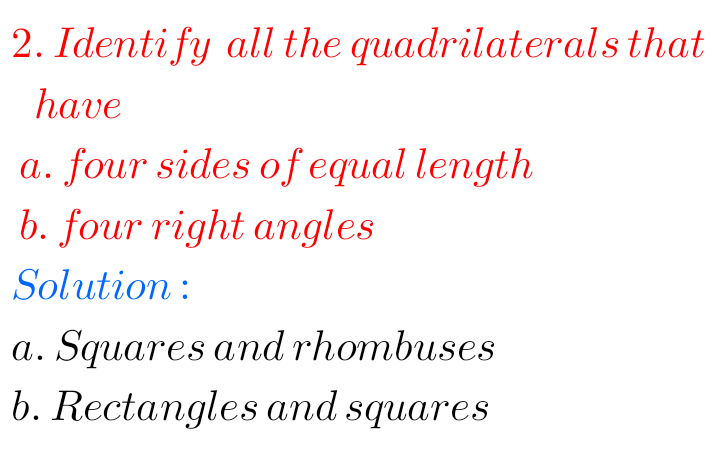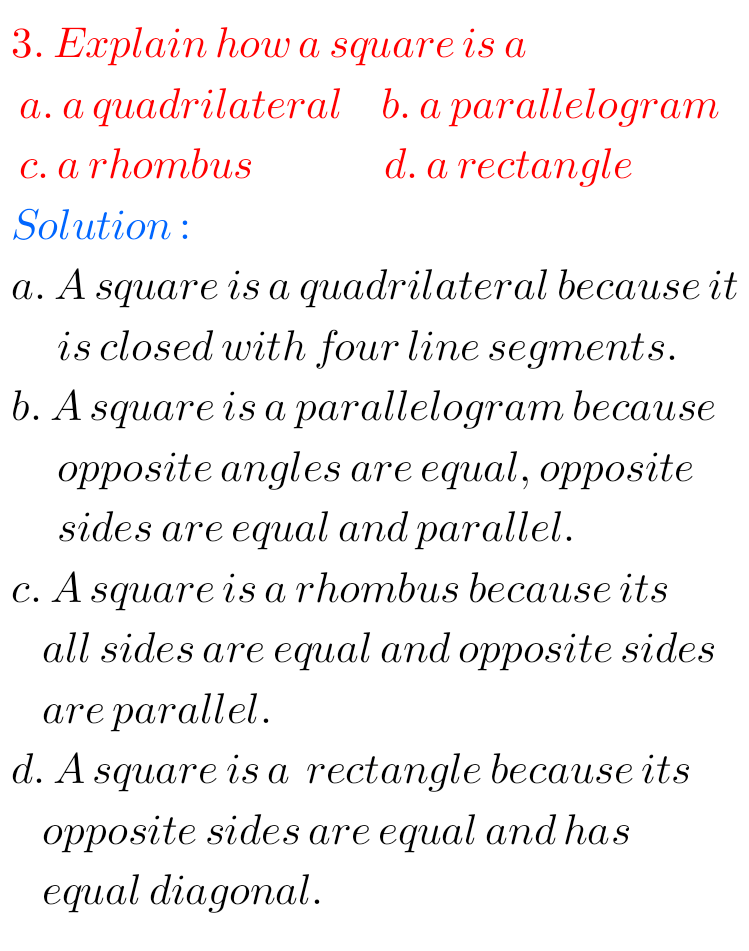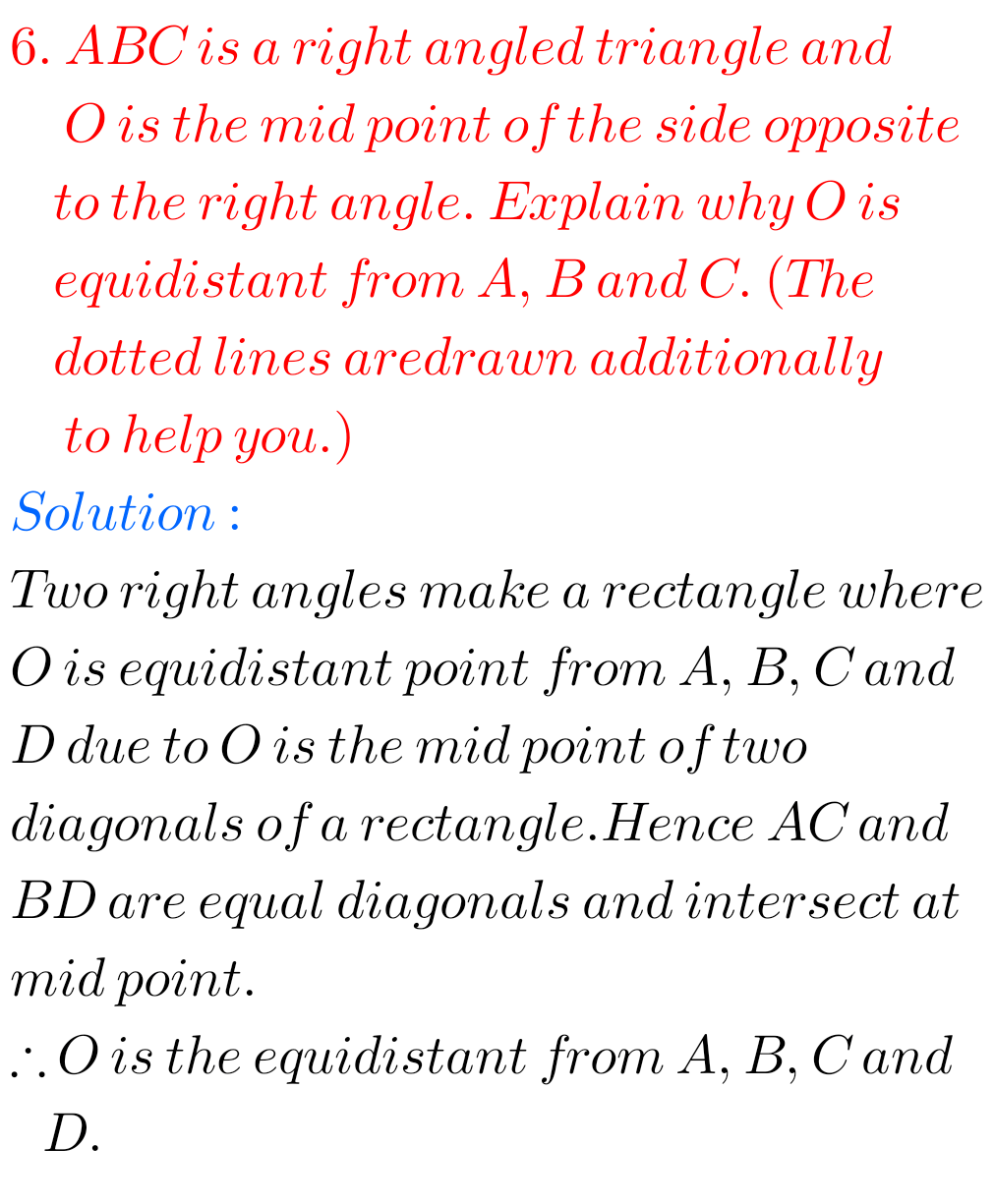# Understanding Quadrilaterals Exercise 3.4 solutions NCERT maths class 8

NCERT maths class 8 chapter 3 Understanding Quadrilaterals Exercise 3.4 solutions are given.

You should study the textbook lesson Understanding Quadrilaterals very well.

Observe the given below solutions and try them in your own method.

NCERT maths class 8 solutions

Exercise 3.1

## Solutions for maths class 8 exercise 3.4 chapter 3 NCERT

Chapter 3

Exercise 3.4### NCERT Maths class 8 Understanding Quadrilaterals exercise 3.4 solutionsNote : Observe the solutions and try them in your own method.

NCERT maths class 7 solutions

NIOS Maths 311 book 2 solutions for some chapters

Inter Maths Solutions

SSC Maths solutions class 10

NCERT maths solutions class 6